STONEHENGE TUTORIALS

We'll now deal with a batch of mounds that relate to Stonehenge, in the same way as has been demonstrated for both Bluestonehenge and Woodhenge. There is a huge selection to choose from ranging from groupings at Durrington Downs, The Packway, The Lesser Cursus and Cursus, Winterbourne Stoke, Normanton Downs, North Kite and the Lake Barrows, Wilsford, Lake Down, around Coneybury Henge, Vespasian's Camp, Larkhill and of course The King Barrows. These several hundred purpose-built, outlying marker positions are situated from less than half a mile, up to 2 or 3 miles out from the 3 main circle sites under study. Stonehenge, Bluestonehenge and Woodhenge act as hub positions for readings onto the outlying surveying markers.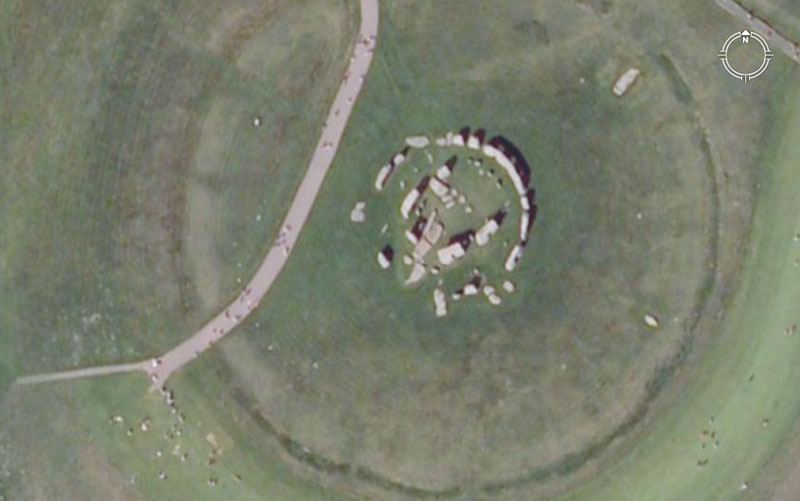A satellite view of Stonehenge.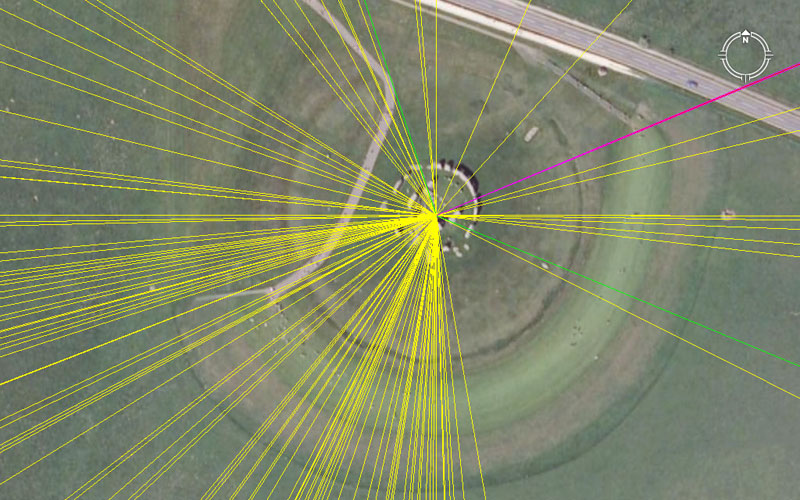Lines fan out from Stonehenge's centre onto outlying mound positions. Each line shown is coded by way of both distance and angle and produces the selfsame factorable values that have been used since time immemorial by European-Caucasoid cousin nations for the maintenance of their civilisations. The lengths found marked into the terrain around Stonehenge can be converted into ancient Babylonian-Sumerian, Egyptian, Greek, Roman, British, Scandinavian or Teutonic, etc., measurement standards, which were all ratio expressions of each other and shared universally amongst the Caucasoid civilisations from remote antiquity. The selfsame, highly factorable numbers were used in measuring out volumes of goods sold in the ancient marketplaces or for materials, such as gold and precious gems, sold according to "grain" weight.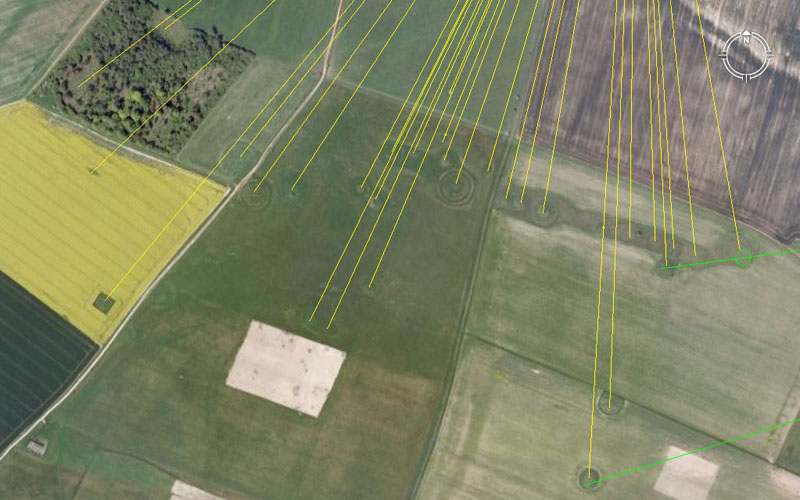The multiple mound site known as Normanton Down sits south of Stonehenge and provides an excellent set of sites for checking coded alignments from Stonehenge. We'll move a bit closer in.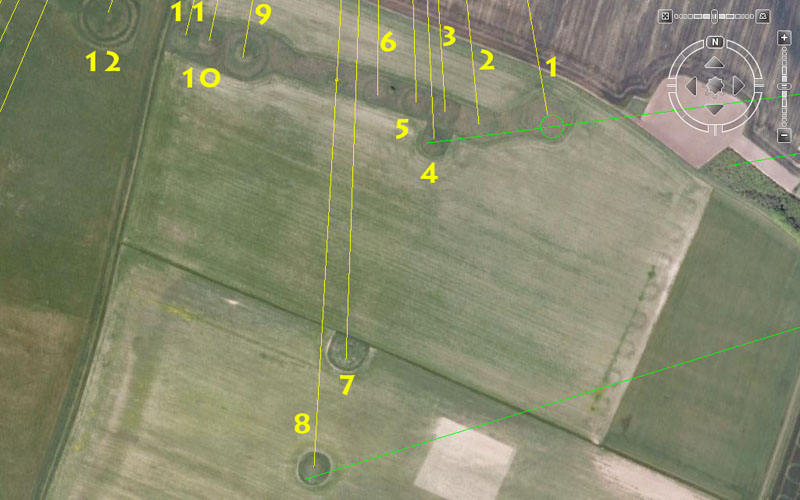The eastern side of the Normanton Downs string of mounds, which also shows two mounds lying slightly further South from the main body. Two green lines are seen to come in from the left to resolve upon these mounds and the lines originate at the hub position of Bluestonehenge. The yellow lines originate at the hub position of Stonehenge.

1. The distance is 3456' and the azimuth angle is 170.1° from the centre of Stonehenge to this mound.

The value 3456 is dynamic navigational coding and the outer rim of Stonehenge's Sarsen Circle was intended to convey 345.6' of circumference, base upon one cross-diameter reading of 110' and using the "11" series form of PI @ 3.141818182. (1728/550ths). There were 30 lintels. each averaging 11.52' of outer face length. If the outer face circumference was considered to be 345.6-miles, then the distance would be 1/72nd of the 24883.2-mile equatorial circumference. this distance extending out to the mound would be 1/37800th of the equatorial circumference of the Earth under the Great Pyramid's literal geodetic assignment.

The angle is encoding a very important lunar value and the sum of 1701-days would be 1/4th of the 6804-day lunar nutation cycle.

1b (Green line). This extends for 5906.25' at an azimuth angle of 82.5°.

The green line extending from Bluestonehenge is in homage to the value 59.0625, which in days would be two lunar months. This overall distance would be 10 times the intended, diagonal face length of the Khafre Pyramid of Egypt.

The angle of 82.5° provides a value used in mathematical progressions related to the "11" series mile of 5280' and 82.5' would be 1/64th of a mile or 5 rods/perches.

2. The distance remains 3456' and the azimuth angle back to Stonehenge is 354.375°.

The degree angle back to Stonehenge codes the lunar year which is 354.375-days.

3. The distance is 3402' and the azimuth angle back to Stonehenge is 356.4°.

Professor Alexander Thom and other surveyors have accepted that the defining diameter of the southern circle at Avebury Henge was 340 feet. The true diameter was intended to convey 340.2 - 3021/5' (radius 170.1'), as this was a special value that related directly to the duration of the lunar nutation cycle (6804-days), where the moon moves from major standstill to minor standstill and back to major standstill. The duration of 3402-days represents the period from major to minor standstill. The value is symbolically encoded (in enlarged form) into the base dimensions of the Khafre Pyramid. It will be remembered that the Khafre base measurement @ 708.75 - 7083/4' per side was 2835' for one complete circumnavigation. In inches this is 34020.

The same coding (340.2'... 324-Greek feet, or 32.4 Hebrew reeds), as found at Avebury Henge, is also found as the defining diameter of the Brodgar Ring in Scotland, as well as a recently excavated post ring site in Germany.

The angle relates to navigation by the mile of 5280 feet and specialised measurements related to the equatorial circumference of the Earth. This distance is 54 chains of 66', 216 rods/perches of 16.5', 648 ancient British fathoms of 5.5' or 5400 links of 7.92". It can also measured in "11" series Egyptian Royal Cubits of 20.625" or the largest Egyptian Royal Cubit @ 20.736" (1.728'.... note: there are 1728 cubic inches in a cubic foot). The mathematical progression derived from 3564, producing values like 89100 can appear complex, but is found on sites across the entire world and is strongly represented at places like Nasca, Peru, amongst the desert geometry. The value 28512 and related values within the mathematical progression show up amidst the Hebrew-Phoenician volume standards.

 SEPPHORIS DRY VOLUME (decimal fractions). SEPPHORIS DRY VOLUME (fractions) 1 Homer….28512 cubic inches equals 1 Homer….28512 cubic inches equals 10 Ephah….2851.20 cubic inches., or 10 Ephah….28511/5th cubic inches., or 30 Seah…..950.40 cubic inches, or 30 Seah…..9502/5ths cubic inches, or 180 Cab….158.4 cubic inches, or 180 Cab….1582/5thscubic inches, or 720 Log….39.6 cubic inches. 720 Log….393/5ths cubic inches.

4. The distance is 3500' and the azimuth angle from Stonehenge is 177.1875°.

As previously stated, The distance can be expressed as 3600 so-called Roman feet and 3600 ancient Swedish feet simultaneously. It is also 1/10th of an ancient Swedish Mil (mile). The same distance would equate to 1000 ancient Swedish Steg. The length converts fluidly into ancient Greek or Assyrian feet-cubits or Hebrew Reeds. It is 2/3rds of a Greek mile, etc., and was put in place on English soil a couple of thousand years before there was any semblance of Greek or Roman civilisations.

The angle is lunar coding and 177.1875-days would be half a lunar year @ 354.375-days.

4b (Green line). This extends for 6378.75' to Bluestonehenge at an azimuth angle of 82.5°.

This length is lunar coding and the sum of 637.875-days would be 1/4th of the 2551.5-day (7.2-lunar years) period monitored and daily tracked on ancient peg boards like the bronze Calendar of Coligny artefact found in Ain, France.

5. The distance is 3360' and the azimuth angle is 178.2°.

The distance relates to navigation and the sum of 33.6' would be 1/3rd of 1-minute of equatorial arc (100.8'). This distance would therefore equate to 1.8-minutes of arc under the Great Pyramid's literal assignment. Incidentally, one internal cross-measure, lintel to lintel, within the slightly elliptical Sarsen Circle at Stonehenge, achieves 100.8' or 1-second of Earth equatorial arc according to the "6&7" (Greek mile) navigational system.

The angle is, once again, a part of the 3564 numerical progression and the "Y" Holes Circle at Stonehenge was set out to have a diameter of 178.2'.

6. The distance is 3333.33333' and the azimuth angle is .672 return to Stonehenge.

The distance is simply 1/3rd of 10,000' The same mound would have, undoubtedly, carried a distance code of 3360' to its southern side to accentuate the navigational code 336-672.

The azimuth angle, only just off due North is in homage to the navigational code or mathematical progression based upon .672, 6.72, 67.2 or 672

ALEXANDRIAN WEIGHT.

1 Talent…….403200.0 grains equals:
60 Mina…….6720.0 grains, or
80 Libra…….5040.0 grains, or
960 Uncia…..420 grains.

7. The distance is 4320' and the azimuth angle is 2.0625° (21/16).

The length is navigational coding and a mathematical progression, based upon 432, produces a string of very important values used to describe the equatorial circumference of the Earth or duration of the Precession of the Equinoxes, etc. For example: the length of 432' would be 1/14th of 1-minute of arc. Similarly, there would be 43.2 double lunar months in the 2551.5-day lunar period monitored within the Sabbatical calendar. The sum of 432-years would represent 1/60th of the 25920-year duration of the Precession of the Equinoxes. The length of the Great Pyramid is 432 Hebrew Royal Cubits of 21" each.

The angle is in homage to the "11" series Egyptian Royal Cubit of 20.625", but the same mound would have offered tutorials concerning the slightly smaller Egyptian Royal Cubit as well. The formula to find the lengths of the cubits was to divide the base length of the Great Pyramid by 440. Therefore: 9072" (756' ... for 1/8th of 1-minute of arc) ÷ 440 = 20.61818182". A Royal Cubit of this length can be seen at the Turin Museum. Under the "11" series navigational system the Great Pyramid was considered to be 756.25' in length (for 1/8th of 1-minute of arc) or 9075". Therefore: 9075 ÷ 440 = 20.625".

If the inch lengths of these Royal Cubits were read as miles (of 5280-feet each) and then multiplied by 1200, then the value derived would represent the equatorial size of the Earth under that navigational system. Therefore: 20.61818182 X 1200 = 24741.8181814 (this same length would be 24883.2 Greek miles of 5250-feet each). Also: 20.625 X 1200 = 24750. For more information on the 3 Egyptian Royal Cubits and what their functions were CLICK HERE

8. The distance is 4725' and the azimuth angle is 3.3333°.

The length is lunar coding and is a value found on the Khafre Pyramid of Egypt. The pyramid was built to the 3,4,5 triangle concept wherein the design height of the edifice was 472.5'. The sum of 472.5-days would be 16 lunar months of 29.53125-days each (Double months = 59.0625-days). This distance at Stonehenge would equate to 4500 Greek feet, or 450 Hebrew reeds.

 TRIDRACHM (BEQA) SYSTEM (Decimal fractions). TRIDRACHM (BEQA) SYSTEM (fractions). 1 Talent………590625 grains, equals: 1 Talent………590625 grains, equals: 60 Mina…….. @ 9843.75 grains, or 60 Mina…….. @ 98433/4 grains, or 3000 Tridrachm.. @ 196.875 grains. 3000 Tridrachm.. @ 1967/8 grains.

 DOUBLE-BEQA SYSTEM (Decimal fractions). DOUBLE-BEQA SYSTEM (fractions). 1 Libra……… 4725 grains, equals: 1 Libra……… 4725 grains, equals: 12 Uncia……. @ 393.75 grains, or 12 Uncia……. @ 3933/4grains, or 24 Semuncia.. @ 196.875 grains. 24 Semuncia.. @ 1967/8 grains.

The angle is simply based upon dividing 10 by 3. The angle could also be read as 3.36, which would provide a base value in a navigational mathematical progression. The sum of 33.6' would be 1/3rd of 1-second of equatorial arc.

8b. The distance is 3281.25' and the azimuth angle is 3.33333°.

The length is lunar coding and 3.28125-days would be 1/9th of a lunar month. The value provides a string of both lunar and navigational values when used in a mathematical progression. The length of 328.125' (337.5 Roman overland feet of 11.666666").would be would be 1/16th of a Greek mile. Note: The Roman equatorial circumference of the Earth value was based upon 75-Roman miles per degree of arc. Their mile was 5000 Roman feet and, using the 11.666666-inch navigational foot, this equated to 25000 Greek miles @ 5250-feet each or 131250000 British feet (135000000 Roman feet) for the total circumnavigation of the globe. That means: 131250000 British feet ÷ 4 = 32812500'. This distance of 3281.25' at Stonehenge is, therefore, 1/40000th of the equatorial circumference. under the navigational system used by the Romans.

8c (Green Line). This line extends for 7087.5' at an azimuth angle of 72.9°.

This linking line from Bluestonehenge to Normanton Downs carries the same lunar-distance code as linking lines from Bluestonehenge to both Stonehenge and Woodhenge. It is also the same coding as the line from the obelisk position at Avebury henge to the doorway position at West Kennet Longbarrow (where the return azimuth angle is 354.375° ... half of 708.75). This distance at Stonehenge would be 10-times the design length of the Khafre Pyramid.

The azimuth angle is also dynamic lunar and navigational coding simultaneously. The sum of 72.9-days would be 1/35th of the 2551.5-day period of the moon monitored within the lunisolar Sabbatical Calendar. The Bush Barrow Lozenge gold artefact, found in a tumulus grave near Stonehenge, has a design width of 7.29". The sum of 7.29" would be 1/8th of a Roman lunar pace (58.32"). Note: The Romans used two very close proximity variations on their "foot" in much the same way as the Egyptians did with their Royal Cubits. The Roman overland foot was 11.666666" (pace: 58.333333") whereas their "lunar" or volume capacity "foot" was 11.664" (pace: 58.32").

9. The distance is 3240' and the azimuth angle is 9.6°.

The length relates to the duration of the Precession of the Equinoxes and 3240 years equals 1/8th of that period. A mathematical progression based upon 324 also produces a string of very useful navigational values within the "6&7" navigational system.

The angle value, when used in a mathematical progression, also produces dynamic navigational values. There would, for example, be 960 ancient fathoms of 5.5' in a mile of 5280'.

10. The distance is 3200' and the azimuth angle is 12°.

A 360° compass system is fluidly divisible by 32 and the traditional way to divide the compass is by halves, quarters, eighths, sixteenths and thirty-second Many ancient compass circle designs show 32 divisions. The ancient rod/perch measurement (16.5') is 1/320th of a mile. A 64-square matrix (8 X 8 or 32 X 2) was very important for tracking daily positions of the Sun and Moon and the Calendar of Coligny, comprising 128 half squares or 64 full squares, was an ancient European example of a lunisolar Sabbatical Calendar. The chessboard, out of Egypt, was undoubtedly a calculation matrix prior to the advent of the game of chess.

The angle can also relate to the sexagesimal method by which the 360° compass is set out into degrees, seconds and minutes of arc. The same conception holds true for our chronology system, which has been handed down and used continuously since ancient times and are now attributed to the Sumerians.

11. The distance remains 3200' and the azimuth angle is 13.608°.

The angle relates to the duration of the lunar nutation cycle of 6804-days, wherein 1360.8-days would be 1/5th of the period. A mathematical progression based upon 13.608 would deliver up a whole string of useful values related to that particular lunar cycle. Likewise, there would be 18.75 instances of 136.08-days in the 2551.5-day period of the moon (7.2 lunar years) monitored and daily tracked within the Sabbatical Calendar system.

12. The distance is 3072' and the azimuth angle is 16.2°.

Whereas the ancient formula for determining the size of the Earth was 12 X 12 X 12 X 12 X 1.2 = 24883.2, other mnemonic, calibrated circle devices existed also. One could use the value 307.2 X 3 X 3 X 3 X 3 = 24883.2. The incremental value of 307.2-miles would work exceptionally well in a circle set out in ninths of the equatorial circumference, where each ninth division was further subdivided into ninths. Therefore: 307.2-miles X 81 (9 X 9) = 24883.2.

The circumference of the Earth could be scaled down and represented in a circle divided into 360° segments or 320-parts. An example of a 360°, scaled down circle is the outer rim of the Sarsen Circle at Stonehenge, where the rim circumference was intended to be 345.6' or 30 lintels each with a length of 11.52'. Therefore 345.6' = 360 X 11.52". At the same time, 307.2' = 320 X 11.52". The 1152 value is highly important to navigation and readings of the equatorial size of the Earth and occurs copiously in ancient mathematics. An example of this is: 6048' (1-minute of arc according to the Great Pyramid's literal geodetic assignment) = 11.52 X 525' (1 short Greek Stadia, or 50 Hebrew reeds, or 500 Greek feet or 1/10th of a Greek mile).

The angle reading is in homage to rounded PHI (1.62 to 1 as compared to 1.6180339 to 1). Just as the Greek half Stadia of 315' was in deference to PI and a rounded form of the PI ratio, so was 162 a rounded form of the PHI ratio. By using these values that were in very close proximity to the true ratios, dimensions within buildings could be read according to either PHI or PI values or positional plotting at sea was facilitated in converting circle diameters to circumferences or vice-versa. The value 162 (81 X 2) is highly important to lunar progressions and 81-days X 315 = 25515-days or 72 lunar years. Alternatively, 16.2-days X 420 = 6804 (the duration of the lunar nutation cycle).13. The distance is 3090.2' and the azimuth angle back to Stonehenge is 21°.

The distance code his homing in on the PHI reciprocal: 1 ÷ 1.6180339 (PHI) = .61804 and 3090.2' is half of 6180.4'. As it turns out, this value was amongst the most important of antiquity and the PHI reciprocal formula was the basis for the fabrication of the "Volume" vessels used in the ancient marketplaces of the cousin Caucasoid nations. This was the formula that ancient mathematicians used for "squaring the circle". To understand how this formula worked in providing perfect, code bearing volume vessels, CLICK HERE

The angle accentuated the value 21 and both the Celtic & Hebrew Royal Cubits were 21" in length. There would be 3000 such Royal Cubits in a Greek mile of 5250-feet. Based upon the fact that Royal Cubits were generally 1.166666666 in size large than their common cubit counterparts, the 21" Cubit would also have represented the larger expression of the common cubits of 18". By consequence, the 21" cubit was 1.8 to 1 larger than the Roman overland foot of 11.66666", so conversions between the systems could be accomplished by simple ratio.

14. The distance is 3000' and the azimuth angle is 21.875°.

The distance related to the sexagesimal system of calibration,

The angle value, when used in a mathematical progression, produces a string of values that are very important to lunar and navigational calculations.

14b. The distance is 2953.125' and the azimuth angle remains 21.875°.

This length is in homage to the lunar month @ 29.53125-days.

15. The distance is 3125' and the azimuth angle is 24.3°.

This length relates to the ancient British league (16500'), which was 3.125 British miles. The value 3.125 was used as one of several close proximity forms of PI and pressed into service for particular calculations.

The angle code is lunar. There would be 105 intervals of 24.3-days (583.2-hours) in the 2551.5-day lunar period of the Sabbatical Calendar and 280 intervals in the 6804-day lunar nutation cycle. Note: Because the ancient Hebrew reed was 10.5' and the Roman lunar pace was 58.32", staffs calibrated to both the pace and the half reed would have acted as lunar calculators in the hands of adept mathematicians. The most convenient or fluidly versatile lunar calculator would be the half reed at 5.25' (63").

16. The distance is 3168' and the azimuth angle is 26.4°.

This length is .6 of a British mile.

The angle is also in homage to the British mile and 2 X 2640' = 5280'.

17. The distance is 3437.5' and the azimuth angle is 27.5°.

The distance is in homage to 1° of arc on the Equatorial circumference, which was 68.75-miles (of 5280') under the 24750-mile assignment and 34.375-miles X 2 = 68.75.

The angle value provides a dynamic mathematical progression related to the mile of 5280' and the equatorial circumference of 24750-miles or navigation by the "11" family of numbers.

18. The distance is 3456' and the azimuth angle is 25.78125.

The length value is ten times the intended circumference of the outer rim of the Sarsen Circle at Stonehenge and related to the equatorial size of the Earth. This distance out to the mound position would be 1/37800th of the equatorial circumference under the literal system encoded into the base dimensions of the Great Pyramid. The length would be 2000 of the largest Egyptian Royal Cubits @ 20.736" (1.728').

The angle provides a mathematical progression related to the 24750-mile equatorial circumference and 25.78125-miles would be 1/960th part of that circuit.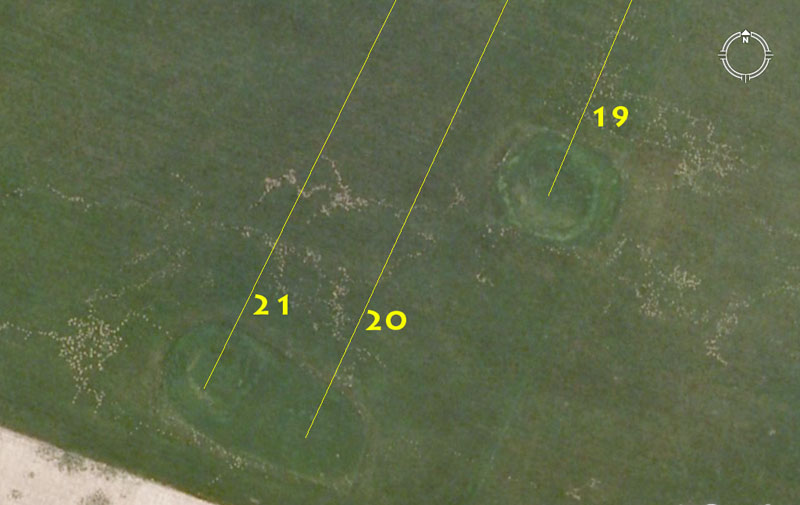19. The distance is 3937.5 and the azimuth angle is 23.04°.

This length is both lunar and navigational coding simultaneously and 39.375-days would be 1/9th of a lunar year.

The angle value is part of the 11.52 progression that relates strongly to navigation and the square footage area of each face of the Great Pyramid was 8-Egyptian acres of 28800 square feet each or 230400-square feet. This was based upon a base length of 756' a top altar length of 44' and a face length linking the two of, essentially, 576'.

20. The distance is 4252.5' and the azimuth angle is 24.75°.

The length is lunar and relates to the duration of the lunar nutation cycle of 6804-days. The sum of 425.25-days would be 1/16th of that period. Alternatively the sum of 425.25-days would be 1/6th of the 2551.5-day period of the moon monitored within the lunisolar Sabbatical Calendar.

21. The distance remains 4252.5' and the azimuth angle is 26.25°.

The angle is both strong lunar and navigational coding simultaneously. There would be 13.5 intervals of 26.25-days in a lunar year and 26.25-feet equates to 1/200th of a Greek mile of 5250'.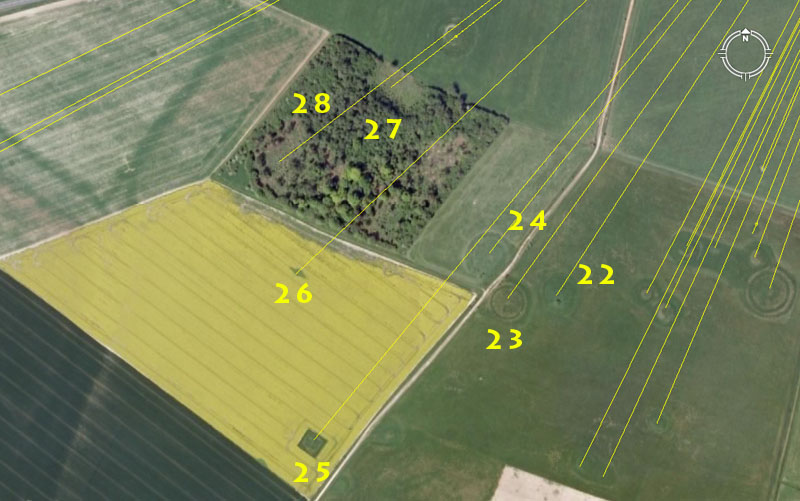22. The distance is 3645' and the azimuth angle is 33°.

This length is lunar coding and there would be 7 intervals of 364.5-days in 2551.5-days (7.2 lunar years). The sum of 3645' is also 750 Roman lunar paces of 58.32" each or 3750 Roman lunar feet or 11.664" each. The distance would also have been read and interpreted in Roman overland feet of 11.66666" each.

The angle is navigational coding using the mile of 5280-feet and 33' would be half a chain or 1/160th of a mile.

23. The distance is 3780' and the azimuth angle is 35.64°.

This distance is 5/8ths of 1-minute of equatorial arc according to the Great Pyramid's literal geodetic assignment.

The angle provides a mathematical progression for reading the size of the Earth as 24750-miles. The "Y" Holes Circle at Stonehenge was calibrated to have a diameter width of 178.2' or 2.475' X 72.

24. The distance is 3666.66666' and the azimuth angle is 38.5°.

This distance is 1/3rd of 11000'.

The angle relates to the value 77 (twice 38.5) and is an "11" series progression. It's highly likely that the same mound site would have coded the angle of 218.75° from Stonehenge and a progression based upon that angle would relate to lunar, navigational and septimal divisions simultaneously.

25. The distance is 4800' and the azimuth angle is 39.6°.

The 48 value is dynamic in a mathematical progression to do with navigation.

The 39.6 value relates to the equatorial diameter of the Earth, wherein the sum of 3960-miles would be the radius.

26. The distance is 4320' and the azimuth angle is 46.656°.

As stated earlier, the length is navigational and equates to 51840". The 432 value featured in mathematical progressions that related to navigation and the Precession of the Equinoxes. The Great Pyramid @ 756', measured in Hebrew Royal Cubits of 21", was 432 cubits per side or 1728 for one circumnavigation (note: there are 1728 cubic inches in a cubic foot).

The 46.656 value is dynamic navigational coding and was strongly represented at Stonehenge by a marker that sat on the Avenue at 45° azimuth. That marker sat at an intended distance of 233.28' from Stonehenge's centre, thus marking the edge of a circle on the site that had a diameter of 466.56. The circle was the outermost in a set that reduced by PHI. To see the PHI reducing circles and squares at Stonehenge CLICK HERE

A mathematical progression based upon 46.656 delivers up fractions of the Earth's equatorial circumference given in feet. Therefore: 12 X 12 X 12 X 12 X 1.2 X 5250' = 130636800' ÷ 46656 = 2800. Essentially, this was a way of calibrating the equatorial circumference into 28 or 56 positions for septimal readings within a 360° circuit. Note: there were 56 post positions on the Aubrey Circle at Stonehenge.

The doubled value of 46656 is 93312 and these two numbers were used as the basis of the Sumerian-Babylonian Royal Standard for weight.

 ROYAL SINGLE STANDARD (decimal fractions). ROYAL SINGLE STANDARD (fractions). 1 Talent……… 466560 grains, equals: 1 Talent……… 466560 grains, equals: 60 Mina……… @ 7776 grains, or: 60 Mina……… @ 7776 grains, or: 3600 Shekel….. @ 129.6 grains. 3600 Shekel….. @ 129.6 grains.

 ROYAL DOUBLE STANDARD (decimal fractions). ROYAL DOUBLE STANDARD (fractions). 1 Talent………933120 grains, equals: 1 Talent………933120 grains, equals: 60 Mina…….. @ 15552 grains, or: 60 Mina…….. @ 15552 grains, or: 3600 Shekel… @ 259.2 grains. 3600 Shekel… @ 2591/5 grains.

Alternatively, the half value of 4665.6 was used in the Greek liquid volume standard.

 GREEK VOLUMES…LIQUID (decimal fractions). GREEK VOLUMES…LIQUID (fractions). 1 Metretes…2332.8 cubic inches equals: 1 Metretes…23324/5 cubic inches equals: 12 Chous…..194.4 cubic inches, or 12 Chous…..1942/5 cubic inches, or 144 Cotyle…16.2 cubic inches, or 144 Cotyle…161/5 cubic inches, or < 576 Oxybaphhon…4.05 cubic inches, or 576 Oxybaphhon…41/20 cubic inches, or 864 Cyathus…2.70 cubic inches. 864 Cyathus…27/10 cubic inches.

27. The distance is 3456' and the azimuth angle is 51.84°.

As stated for position 1: The value 3456 is dynamic navigational coding and the outer rim of Stonehenge's Sarsen Circle was intended to convey 345.6' of circumference, base upon one cross-diameter reading of 110' and using the "11" series form of PI @ 3.141818182. (1728/550ths). There were 30 lintels. each averaging 11.52' of outer face length. If the outer face circumference was read in increased scale as 345.6-miles, then the distance would be 1/72nd of the 24883.2-mile equatorial circumference. This 3456' distance extending out to the mound would be 1/37800th of the equatorial circumference of the Earth under the Great Pyramid's literal geodetic assignment.

27b. The distance is 3090.2' and the azimuth angle remains 51.84°.

As stated for position 13: The distance code his homing in on the PHI reciprocal: 1 ÷ 1.6180339 (PHI) = .61804 and 3090.2' is half of 6180.4'. As it turns out, this value was amongst the most important of antiquity and the PHI reciprocal formula was the basis for the fabrication of the "Volume" vessels used in the ancient marketplaces of the cousin Caucasoid nations. To understand how this formula worked in providing perfect, code bearing volume vessels, CLICK HERE

With regards to the angle, it is the same as what has been stated for position 4 out from Woodhenge: The Great Pyramid's slope angle was coded to be 51.84°. The Great pyramid @ 72 reeds per base side equals 5184 reeds of area covered by the edifice. The ancient value used to describe the duration of the Precession of the Equinoxes was 25920-years (360 X 72). This equates to 5184-years X 5. When the length of 1 ancient British league (16500' or 3.125-miles) is used as the diameter of a circle, it produces a circumference of 51840' using the special rendition of PI that applied to all "11" series numbers (1728/550ths ... 3.141818182). A league of travel therefore produced a circumference value that was perfectly divisible within a 360° compass environment. This was the basis of positional plotting at sea, where the degree angle back to the point of departure or onwards to the destination could always be calculated with accuracy, despite many course changes while tacking by the wind. The 1728/550ths navigational principle is encoded into the diameter and circumference of Silbury Hill near Avebury Henge in one cross-measure. The azimuth angle that the Octagon geometric earthmound complex of Newark, Ohio, USA lies on is 51.84°. The sum of 51.84 X 250 = 12960, which was the volume, in cubic inches, of the ancient Babylonian Homer.

 GREEK CAPACITY MEASURES…DRY (Decimal fractions). GREEK CAPACITY MEASURES…DRY (Fractions). 1 Medimnus…3110.4 cubic inches, equals: 1 Medimnus…3110.2/5 cubic inches, equals: 6 Hecteus…518.40 cubic inches, or 6 Hecteus…518.2/5 cubic inches, or 48 Choenix…64.80 cubic inches, or 48 Choenix…64.4/5 cubic inches, or 192 Coytle…16.20 cubic inches, or 192 Coytle…16.1/5 cubic inches, or 1152 Cyathus…2.70 cubic inches. 1152 Cyathus…2. 7/10 cubic inches.

28. The distance is 4050' and the azimuth angle is 52.5°.

As stated for position 14 out from Woodhenge: The length is in homage to the value 405, which featured strongly in lunar calculations. For example, there would be 168 X 40.5-days in the 6804-day cycle of the Moon (note: there are 168-hours in a week). There are 63 periods of 40.5-days in the 2551.5-day (7.2-lunar years) monitored in the lunisolar Sabbatical Calendar.

 GREEK VOLUMES…LIQUID (decimal fractions). GREEK VOLUMES…LIQUID (fractions). 1 Metretes…2332.8 cubic inches equals: 1 Metretes…23324/5 cubic inches equals: 12 Chous…..194.4 cubic inches, or 12 Chous…..1942/5 cubic inches, or 144 Cotyle…16.2 cubic inches, or 144 Cotyle…161/5 cubic inches, or 576 Oxybaphhon…4.05 cubic inches, or 576 Oxybaphhon…41/20 cubic inches, or 864 Cyathus…2.70 cubic inches. 864 Cyathus…27/10 cubic inches.

The azimuth angle is, of course, coding the 5.25 mathematical progression, which was at the basis of the Greek overland measurement system and found positively encoded into the British landscape thousands of years before there was a Greek civilisation.

The foregoing provides a brief glimpse of the length and angle codes that were carefully built into the ancient British landscape around Stonehenge. The sample given, however, should be sufficient for the researcher, and more especially those with a background in surveying or ancient metrology, to see that there is nothing haphazard about the outer mound layout. Everything was purpose placed for severe and grueling tutorials for navigator-astronomers, whose responsibility it would become to guide sailing ships, their crews and precious cargos across the vast oceans of the world.

Although for the purposes of this exercise, generally only one distance and one angle code is given for an outer-marker mound. Often, however, where the opportunity was present, two or more close proximity codes could be placed, by marked positions, on a single mound. Frequently, an angle away from the hubstone at Stonehenge, Woodhenge or Bluestonehenge could carry an altogether different numerical code in the return angle (180°-opposed). An example of this might be, say, an angle of 21.6° (Precession of the equinoxes code ... the sun spends 2160-years in each house of the zodiac during the 25920-year cycle of Precession) out to a mound. This would produce a return angle of 201.6° (code to do with the equatorial size of the Earth, wherein 2016' would be 1/3rd of 1-minute of arc).

The overall point is: Our highly paid academics have, for far too long, let their prejudices and apparent lack of proper surveying training, distort the real truth regarding our European ancestors and what the ancient astronomer-surveyors were doing when building these interrelating complexes of monuments. Although Dr. Michael Parker-Pearson and his team's discovery of Bluestonehenge is to be applauded, his new "bone-yard" hypothesis to explain the purpose of Stonehenge-Bluestonehenge is abysmal scholarship in consideration of the easy to detect mathematical encoding of the surrounding landscape. Our "experts" once again, attempt to reduce high-minded mathematician-scientists of 5000-years ago to the lowest common denominator.

In the absence of vast resources of paper to produce "perishable" books, the ancient savants recorded their scientific data in the positions of huge, "eternal" obelisks or marker mounds that stood off from a central hubstone. All that was required to extract the profound scientific principles from the landscapes was accurate measuring rules and a 360° compass system, coupled with the profound mathematical knowledge of a "Master of the Craft" tutor-overseer. Without this advanced scientific knowledge the concept of abundant, functioning, intercooperative society or nationhood was nigh on impossible to achieve and the great civilisations of antiquity would not have blossomed.

To see this selfsame encoding of the sciences into the Avebury Henge landscape, situated about 20-miles to the North of Stonehenge CLICK HERE

Martin Doutré ©

November 17th 2009.

To be continued.